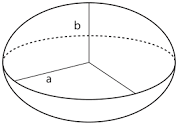## How to Calculate and Solve for the Area of an Ellipsoid | The Calculator EncyclopediaThe image above is an ellipsoid.

To compute the area of an ellipsoid, three essential parameters are needed and this parameters are axis (a)axis (b) and axis (c).

The formula for calculating the area of an ellipsoid:

A = 4π((ab)1.6 + (ac)1.6 + (bc)1.63)1 / 1.6

Where;
A = Area of the ellipsoid
a = Axis of the ellipsoid
b = Axis of the ellipsoid
c = Axis of the ellipsoid

Let’s solve an example;
Find the the area of an ellipsoid when the axis (a) of the ellipsoid is 12 cm, axis (b) of the ellipsoid is 6 cm and axis (c) of the ellipsoid is 2 cm.

This implies that;
a = Axis of the ellipsoid = 12 cm
b = Axis of the ellipsoid = 6 cm
c = Axis of the ellipsoid = 2 cm

A = 4π((ab)1.6 + (ac)1.6 + (bc)1.63)1 / 1.6
A = 4π(((12)(6))1.6 + ((12)(2))1.6 + ((6)(2))1.63)1 / 1.6
A = 4π(((72)1.6 + (24)1.6 + (12)1.6)3)1 / 1.6
A = 4π(((936.98) + (161.56) + (53.29))3)1 / 1.6
A = 4π((1151.84)3)1 / 1.6
A = 4π(383.946)1 / 1.6
A = 4π(383.946)0.625
A = 4π(41.2258)
A = (12.566)(41.2258)
A = 518.059

Therefore, the area of the ellipsoid is 518.059 cm2.

## How to Calculate and Solve for the Axis and Volume of an Ellipsoid | The Calculator EncyclopediaThe image above is an ellipsoid.

To compute the volume of an ellipsoid, three essential parameters are needed and this parameters are axis (a)axis (b) and axis (c).

The formula for calculating the volume of an ellipsoid:

V = 4πabc3

Where;

V = Volume of the ellipsoid
a = Axis of the ellipsoid
b = Axis of the ellipsoid
c = Axis of the ellipsoid

Let’s solve an example;
Given that the axis of the ellipsoid (a) is 9 cm and axis of the ellipsoid (b) is 11 cm with the axis (c) of 14 cm. Find the volume of the ellipsoid?

This implies that;
a = Axis of the ellipsoid = 9 cm
b = Axis of the ellipsoid = 11 cm
c = Axis of the ellipsoid = 14 cm

V = 4πabc3
V = 4π(9 x 11 x 14)3
V = 4π(1386)3
V = (12.56)(1386) / 3
V = (17416.98967) / 3
V = 5805.66

Therefore, the volume of the ellipsoid is 5805.66 cm3.

Calculating the Axis (a) of an Ellipsoid using the Volume of the Ellipsoid, Axis (b) of the Ellipsoid and Axis (c) of the Ellipsoid.

a = V3 / 4πbc

Where;
a = Axis of the ellipsoid
V = Volume of the ellipsoid
b = Axis of the ellipsoid
c = Axis of the ellipsoid

Let’s solve an example;
Find the axis (a) of an ellipsoid when the volume of the ellipsoid is 280 cm3 with an axis (b) of 18 cm and axis (c) of 8 cm.

This implies that;
V = Volume of the ellipsoid = 280 cm3
b = Axis of the ellipsoid = 18 cm
c = Axis of the ellipsoid = 8 cm

a = V3 / 4πbc
a = 280 x 3 / (12.566)(18 x 8)
a = 840 / (12.566)(144)
a = 840 / 1809.504
a = 0.46

Therefore, the axis (a) of the ellipsoid is 0.46 cm.